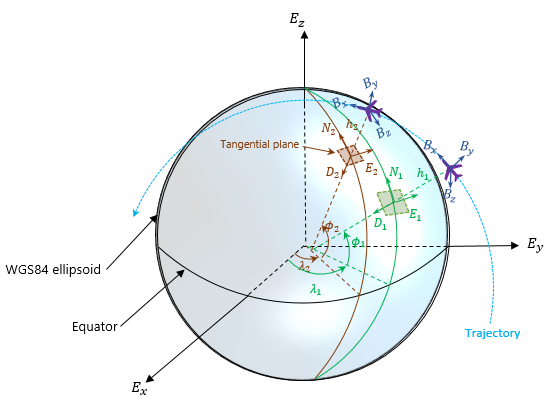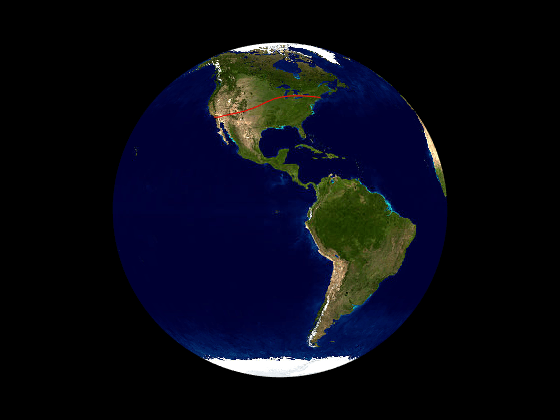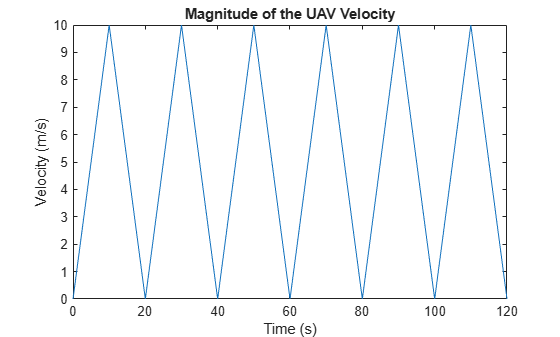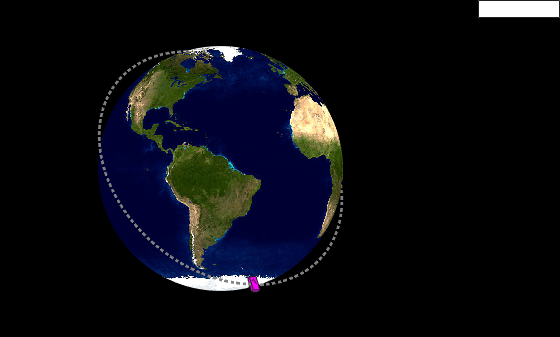Main Content

## Model Platform Motion Using Trajectory Objects

This topic introduces how to use three different trajectory objects to model platform trajectories, and how to choose between them.

### Introduction

Sensor Fusion and Tracking Toolbox™ provides three System objects that you can use to model trajectories of platforms including ground vehicles, ships, aircraft, and spacecraft. You can choose between these trajectory objects based on the available trajectory information and the distance span of the trajectory.

• `waypointTrajectory` — Defines a trajectory using a few waypoints in Cartesian coordinates that the trajectory must pass through. The trajectory assumes the reference frame is a fixed North-East-Down (NED) or East-North-Up (ENU) frame. Since the trajectory interpolation assumes that the gravitational acceleration expressed in the trajectory reference frame is constant, `waypointTrajectory` is typically used for a trajectory defined within an area that spans only tens or hundreds of kilometers.

• `geoTrajectory` — Defines a trajectory using a few waypoints in geodetic coordinates (latitude, longitude, and altitude) that the trajectory must pass through. Since the waypoints are expressed in geodetic coordinates, `geoTrajectory` is typically used for a trajectory from hundreds to thousands of kilometers of distance span.

• `kinematicTrajectory` — Defines a trajectory using kinematic properties, such as acceleration and angular acceleration, expressed in the platform body frame. You can use `kinematicTrajectory` to generate a trajectory of any distance span as long as the kinematic information of the trajectory is available. The object assumes a Cartesian coordinate reference frame.

The two waypoint-based trajectory objects (`waypointTrajectory` and `geoTrajectory`) can automatically calculate the linear velocity information of the platform, but you can also explicitly specify the linear velocity using the `Velocity` property or a combination of the `Course`, `GroundSpeed`, and `ClimbRate` properties.

The trajectory of a platform is composed of rotational motion and translational motion. By default, the two waypoint-based trajectory objects (`waypointTrajectory` and `geoTrajectory`) automatically generate the orientation of the platform at each waypoint by aligning the yaw angle with the path of the trajectory, but you can explicitly specify the orientation using the `Orientation` property. Alternately, you can use the `AutoPitch` and `AutoBank` properties to enable automatic pitch and roll angles, respectively. For `kinematicTrajectory`, you need to use the `Orientation` property and the angular velocity input to specify the rotational motion of the trajectory.

### `waypointTrajectory`

The `waypointTrajectory` System object defines a trajectory that smoothly passes through waypoints expressed in Cartesian coordinates. Generally, you can use `waypointTrajectory` to model vehicles travelling within hundreds of kilometers. These vehicles include automobiles, surface marine craft, and commercial aircraft (helicopters, planes, and quadcopters). You can choose the reference frame as a fixed ENU or NED frame using the `ReferenceFrame` property. For more details on how the object generates the trajectory, see the Algorithms section of `waypointTrajectory`.

#### `waypointTrajectory` Example for Aircraft Landing

Define the trajectory of a landing aircraft using a `waypointTrajectory` object.

```waypoints = [-421 -384 2000; 47 -294 1600; 1368 174 1300; 995 1037 900; -285 293 600; -1274 84 350; -2328 101 150; -3209 83 0]; timeOfArrival = [0; 16.71; 76.00; 121.8; 204.3; 280.31; 404.33; 624.6]; aircraftTraj = waypointTrajectory(waypoints,timeOfArrival);```

Create a `theaterPlot` object to visualize the trajectory and the aircraft.

```minCoords = min(waypoints); maxCoords = max(waypoints); tp = theaterPlot('XLimits',1.2*[minCoords(1) maxCoords(1)], ... 'YLimits',1.2*[minCoords(2) maxCoords(2)], ... 'ZLimits',1.2*[minCoords(3) maxCoords(3)]); % Create a trajectory plotter and a platform plotter tPlotter = trajectoryPlotter(tp,'DisplayName','Trajectory'); pPlotter = platformPlotter(tp,'DisplayName','Aircraft');```

Obtain the Cartesian waypoints of the trajectory using the `lookupPose` object function.

```sampleTimes = timeOfArrival(1):timeOfArrival(end); numSteps = length(sampleTimes); [positions,orientations] = lookupPose(aircraftTraj,sampleTimes); plotTrajectory(tPlotter,{positions}) axis equal```

Plot the platform motion using an airplane mesh object.

```mesh = scale(rotate(tracking.scenario.airplaneMesh,[0 0 180]),15); % Exaggerated scale for better visualization view(20.545,-20.6978) for i = 1:numSteps plotPlatform(pPlotter,positions(i,:),mesh,orientations(i)) % Uncomment the next line to slow the aircraft motion animation % pause(1e-7) end ```

In the animation, the yaw angle of the aircraft aligns with the trajectory by default.

Create a second aircraft trajectory with the same waypoints as the first aircraft trajectory, but set its `AutoPitch` and `AutoBank` properties to `true`. This generates a trajectory more representative of the possible aircraft maneuvers.

```aircraftTraj2 = waypointTrajectory(waypoints,timeOfArrival, ... 'AutoPitch',true, ... 'AutoBank',true);```

Plot the second trajectory and observe the change in aircraft orientation.

```[positions2,orientations2] = lookupPose(aircraftTraj2,sampleTimes); for i = 1:numSteps plotPlatform(pPlotter,positions2(i,:),mesh,orientations2(i)); % Uncomment the next line to slow the aircraft motion animation % pause(1e-7) end ```Visualize the orientation differences between the two trajectories in angles.

```distance = dist(orientations2,orientations); figure plot(sampleTimes,distance*180/pi) xlabel('Time (sec)') ylabel('Angular Ditance (dge)') title('Orientation Difference Between Two Waypoint Trajectories')```### `geoTrajectory`

The `geoTrajectory` System object generates a trajectory using waypoints in a similar fashion as the `waypointTrajectory` object, but it has two major differences in how to specify waypoints and velocity inputs.

• When specifying waypoints for `geoTrajectory`, express each waypoint in the geodetic coordinates of latitude, longitude, and altitude above the WG84 ellipsoid model. Using geodetic coordinates, you can conveniently specify long-range trajectories, such as airplane flight trajectory on a realistic Earth model.

• When specifying the orientation and velocity information for each waypoint, the reference frame for orientation and velocity is the local NED or ENU frame defined under the current trajectory waypoint. For example, the N1-E1-D1 frame shown in the figure is a local NED reference frame.In the figure,

• Ex, Ey, and Ez are the three axes of the Earth-centered Earth-fixed (ECEF) frame, which is fixed on the Earth.

• (λ1, ϕ1, h1) and (λ2, ϕ2, h2) are the geodetic coordinates of the plane at the two waypoints.

• (N1, E1, D1) and (N2, E2, D2) are the two local NED frames corresponding to the two trajectory waypoints.

• Bx, By, and Bz are the three axes of the platform body frame, which is fixed on the platform.

#### geo`Trajectory` Example For Flight Trajectory

Load the flight data of a flight trajectory from Los Angeles to Boston. The data contains flight information including flight time, geodetic coordinates for each waypoint, course, and ground speed.

`load flightData.mat`

Create a `geoTrajectory` object based on the flight data.

```planeTraj = geoTrajectory([latitudes longitudes heights],timeOfArrival, ... 'Course',courses,'GroundSpeed',speeds);```

Look up the Cartesian coordinates of the waypoints in the ECEF frame.

```sampleTimes = 0:1000:3600*10; positionsCart = lookupPose(planeTraj,sampleTimes,'ECEF');```

Show the trajectory using the `helperGlobeView` class, which approximates the Earth sphere.

```viewer = helperGlobeView; plot3(positionsCart(:,1),positionsCart(:,2),positionsCart(:,3),'r')```You can further explore the trajectory by querying other outputs of the trajectory.

### `kinematicTrajectory`

Unlike the two waypoint trajectory objects, the `kinematicTrajectory` System object uses kinematic attributes to specify a trajectory. Think of the trajectory as a numerical integration of the pose (position and orientation) and linear velocity of the platform, based on the linear acceleration and angular acceleration information. The pose and linear velocity are specified with respected to a chosen, fixed scenario frame, whereas the linear acceleration and angular velocity are specified with respected to the platform body frame.

#### `kinematicTrajectory` Example For UAV Path

Create a `kinematicTrajectory` object for simulating a UAV path. Specify the time span of the trajectory as 120 seconds.

```traj = kinematicTrajectory('SampleRate',1, ... 'AngularVelocitySource','Property'); tStart = 0; tFinal = 120; tspan = tStart:tFinal; numSteps = length(tspan); positions = NaN(3,numSteps); velocities = NaN(3,numSteps); vel = NaN(1,numSteps); ```

To form a square path covering a small region, separate the UAV trajectory into six segments:

• Taking off and ascending in the z-direction

• Moving in the positive x-direction

• Moving in the positive y-direction

• Moving in the negative x-direction

• Moving in the negative y-direction

• Descending in the z-direction and landing

In each segment, the UAV accelerates in one direction and then decelerates in that direction with the same acceleration magnitude. As a result, at the end of each segment, the velocity of the UAV is zero.

```segSteps = floor(numSteps/12); accelerations = zeros(3,numSteps); acc = 1; % Acceleration for taking off and ascending in the z-direction accelerations(3,1:segSteps) = acc; accelerations(3,segSteps+1:2*segSteps) = -acc; % Acceleration for moving in the positive x-direction accelerations(1,2*segSteps+1:3*segSteps) = acc; accelerations(1,3*segSteps+1:4*segSteps) = -acc; % Acceleration for moving in the positive y-direction accelerations(2,4*segSteps+1:5*segSteps) = acc; accelerations(2,5*segSteps+1:6*segSteps) = -acc; % Acceleration for moving in the negative x-direction accelerations(1,6*segSteps+1:7*segSteps) = -acc; accelerations(1,7*segSteps+1:8*segSteps) = acc; % Acceleration for moving in the negative y-direction accelerations(2,8*segSteps+1:9*segSteps) = -acc; accelerations(2,9*segSteps+1:10*segSteps) = acc; % Descending in the z-direction and landing accelerations(3,10*segSteps+1:11*segSteps) =-acc; accelerations(3,11*segSteps+1:end) = acc;```

Simulate the trajectory by calling the `kinematicTrajectory` object with the specified acceleration.

```for i = 1:numSteps [positions(:,i),~,velocities(:,i)] = traj(accelerations(:,i)'); vel(i) = norm(velocities(:,i)); end```

Visualize the trajectory using `theaterPlot`.

```% Create a theaterPlot object and create plotters for the trajectory and the % UAV Platform. figure tp = theaterPlot('XLimits',[-30 130],'YLimits',[-30 130],'ZLimits',[-30 130]); tPlotter = trajectoryPlotter(tp,'DisplayName','UAV trajectory'); pPlotter = platformPlotter(tp,'DisplayName','UAV','MarkerFaceColor','g'); % Plot the trajectory. plotTrajectory(tPlotter,{positions'}) view(-43.18,56.49) % Use a cube to represent the UAV platform. dims = struct('Length',10, ... 'Width',5, ... 'Height',3, ... 'OriginOffset',[0 0 0]); % Animate the UAV platform position. for i = 1:numSteps plotPlatform(pPlotter,positions(:,i)',dims,eye(3)) pause(0.01) end```Show the velocity magnitude of the UAV platform.

```figure plot(tspan,vel) xlabel('Time (s)') ylabel('Velocity (m/s)') title('Magnitude of the UAV Velocity')```#### `kinematicTrajectory` Example For Spacecraft Trajectory

Use `kinematicTrajetory` to specify a circular spacecraft trajectory. The orbit has these elements:

• Orbital radius ($\mathit{r}$) — 7000 km

• Inclination ($\mathit{i}$) — 60 degrees

• Argument of ascending node ($\Omega$) — 90 degrees. The ascending node direction is aligned with the $\mathit{Y}$-direction.

• True anomaly ($\nu$) — –90 degrees

In the figure, $\mathit{X}$-$\mathit{Y}$-$\mathit{Z}$ is the Earth-centered inertial (ECI) frame, which has a fixed position and orientation in space. $\mathit{x}$-$\mathit{y}$-$\mathit{z}$ is the spacecraft body frame, fixed on the spacecraft. $\stackrel{\to }{\mathit{r}}$ and $\stackrel{\to }{\mathit{v}}$ are the initial position and velocity of the spacecraft.To specify the circular orbit using `kinematicTrajectory`, you need to provide the initial position, initial velocity, and initial orientation of the spacecraft with respect to the ECI frame. For the chosen true anomaly ($\mathit{v}\text{\hspace{0.17em}}$= –${90}^{\circ }$), the spacecraft velocity is aligned with the $\mathit{Y}$-direction.

```inclination = 60; % degrees mu = 3.986e14; % standard earth gravitational parameter radius = 7000e3;% meters v = sqrt(mu/radius); % speed initialPosition = [radius*cosd(inclination),0,-radius*sind(inclination)]'; initialVelocity = [0 v 0]';```

Assume the x-direction of the spacecraft body frame is the radial direction, the z-direction is the normal direction of the orbital plane, and the y-direction completes the right-hand rule. Use the assumptions to specify the orientation of the body frame at the initial position.

`orientation = quaternion([0 inclination 0],'eulerd','zyx','frame');`

Express the angular velocity and the angular acceleration of the trajectory in the platform body frame.

```omega = v/radius; angularVelocity = [0 0 omega]'; a = v^2/radius; acceleration = [-a 0 0]';```

Set a simulation time of one orbital period. Specify a simulation step as 2 seconds.

```tFinal = 2*pi/omega; dt = 2; sampleRate = 1/dt; tspan = 0:dt:tFinal; numSteps = length(tspan);```

Create the spacecraft trajectory. Since the acceleration and angular velocity of the spacecraft remain constant with respect to the spacecraft body frame, specify them as constants. Generate position and orientation outputs along the trajectory by using the `kinematicTrajectory` object.

```traj = kinematicTrajectory('SampleRate',sampleRate, ... 'Position',initialPosition, ... 'Velocity',initialVelocity, ... 'Orientation',orientation, ... 'AngularVelocity',angularVelocity, ... 'Acceleration',acceleration, ... 'AccelerationSource','Property', ... 'AngularVelocitySource','Property'); % Generate position and orientation outputs. positions = NaN(3,numSteps); orientations = zeros(numSteps,1,'quaternion'); for i = 1:numSteps [positions(:,i),orientations(i)] = traj(); end```

Use the `helperGlobeView` class and `theaterPlot` to show the trajectory.

```viewer = helperGlobeView(0,[60 0]); tp = theaterPlot('Parent',gca,... 'XLimits',1.2*[-radius radius],... 'YLimits',1.2*[-radius radius],... 'ZLimits',1.2*[-radius radius]); tPlotter = trajectoryPlotter(tp,'LineWidth',2); pPlotter = platformPlotter(tp,'MarkerFaceColor','m'); legend(gca,'off') plotTrajectory(tPlotter,{positions'}) % Use a cube with exaggerated dimensions to represent the spacecraft. dims = struct('Length',8e5,'Width',4e5,'Height',2e5,'OriginOffset',[0 0 0]); for i = 1:numSteps plotPlatform(pPlotter,positions(:,i)',dims,orientations(i)) % Since the reference frame is the ECI frame, earth rotates with respect to it. rotate(viewer,dt) end```### Summary

In this topic, you learned how to use three trajectory objects to customize your own trajectories based on the available information. In addition, you learned the fundamental differences in applying them. This table highlights the main attributes of these trajectory objects.

Trajectory ObjectPosition InputsLinear Velocity Inputs OrientationAcceleration and Angular Velocity InputsRecommended Distance Span
`waypointTrajectory`Cartesian waypoints expressed in a fixed frame (NED or ENU)

One of these options:

• Automatically generate velocity for a smooth trajectory, by default

• Specify velocity in the fixed frame at each waypoint

• Specify course, ground speed, and climb rate in the fixed frame at each waypoint

One of these options:

• Auto yaw by default, auto pitch by selection, and auto bank by selection

• Specify orientation in the fixed frame

Cannot specify From within tens to hundreds of kilometers
`geoTrajectory` Geodetic waypoints in the ECEF frame

One of the these options:

• Automatically generate velocity for a smooth trajectory, by default

• Specify velocity in the local frame (NED or ENU) for each waypoint

• Specify course, ground speed, and climb rate in the local frame (NED or ENU) for each waypoint

One of these options:

• Auto yaw by default, auto pitch by selection, and auto bank by selection

• Specify orientation in the local frame

Cannot specify From hundreds to thousands of kilometers
`kinematicTrajectory`Initial position expressed in a chosen, fixed frame Only initial velocity in the fixed frameOnly initial orientation in the fixed frame Specify acceleration and angular velocity in the platform body frame Unlimited distance span

## Support

#### Sensor Fusion and Tracking for Autonomous Systems

Download white paper